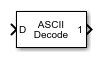# ASCII Decode

Parse ASCII character vector into Simulink values

• Library:
• Simulink Real-Time / RS232

•## Description

The ASCII Decode block parses an input character vector according to a format specifier similar to `scanf` and makes converted values available to the real-time application.

## Ports

### Input

expand all

The input vector can be either 8-bit or 16-bit and signed or unsigned. If the data format is 16-bit, the block ignores the upper 8 bits of each entry.

Data Types: `int8` | `uint8` | `int16` | `uint16`

### Output

expand all

Output ports corresponding to items in Format string.

#### Dependency

Number of variables determines the number of output ports.

Data Types: `double` | `int8` | `uint8` | `int16` | `uint16` | `int32` | `uint32`

## Parameters

expand all

Enter a `scanf` like format string. Each format specifier such as `%d` must match a corresponding part of the input vector. Literal strings in the format must match the first character plus the number of characters. The format specifiers follow the normal description for `scanf`.

An example format string is:

`'alpha %d bravo %f\n'`

#### Programmatic Use

 Block Parameter: `format`

Enter the number of output ports for this block. For example:

If Format string has the value of ```%xmore text%x``` and the input vector for the block has `cdmabcdefgh90`, you must specify the value of the Number of variables parameter as 2.

The first variable is assigned the value `0xcd`. Next, the character vector `mabcdefgh` is considered a match to `more text` because:

• The first character for both character vectors is `m`.

• Both character vectors have the same number of characters.

The second variable is then assigned the value `0x90`. The character vector `mabcdefgh` does not have to match exactly the value of Format string. This behavior is different from the behavior for `scanf`, which requires an exact match.

#### Programmatic Use

 Block Parameter: `nvars`

A cell vector with the same number of elements as specified in Number of variables can specify a different data type for each output port. A single element is replicated. For example:

`nvars=3`

`{ }` — The three outputs are doubles.

`{'uint8'}` — The three outputs are `uint8`.

`{'uint16', 'double', 'uint8'}` — The first output is a `uint16`, the second output is a `double`, and the third output is a `uint8`.

#### Programmatic Use

 Block Parameter: `varids`

## Version History

Introduced in R2020b